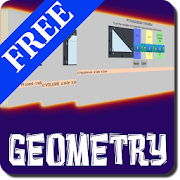# Interactive GeometryEveryone
7
This is a free version of "Interactive Geometry PRO", if you want the ad free version search in the android store for "Interactive Geometry PRO".
This app is ideal for students and graduates, you will find a variety of useful geometry formulas and you can enter a value by touch, the interactive feature make it easy to avoid typing or human errors.
The interface is made in a way that you can see above any variable it's value.
Topics:
"GEOMETRY-RECTANGLE PERIMETER",
"GEOMETRY-RECTANGLE AREA",
"GEOMETRY-TRIANGLE PERIMETER",
"GEOMETRY-TRIANGLE AREA",
"GEOMETRY-PARALLELOGRAM PERIMETER",
"GEOMETRY-PARALLELOGRAM AREA",
"GEOMETRY-TRAPEZOID PERIMETER",
"GEOMETRY-TRAPEZOID AREA",
"GEOMETRY-CIRCLE PERIMETER",
"GEOMETRY-CIRCLE AREA",
"GEOMETRY-CIRCLE SECTOR LENGTH",
"GEOMETRY-CIRCLE SECTOR AREA",
"GEOMETRY-ELLIPSE AREA",
"GEOMETRY-RECTANGULAR PARALLELEPIPED VOLUME",
"GEOMETRY-SPHERE SURFACE",
"GEOMETRY-SPHERE VOLUME",
"GEOMETRY-CYLINDER LATERAL SURFACE",
"GEOMETRY-CYLINDER VOLUME",
"GEOMETRY-CONE VOLUME",
"GEOMETRY-PYRAMID VOLUME",
"GEOMETRY-ELLIPSOID VOLUME",
"GEOMETRY-DISTANCE BETWEEN 2 POINTS",
"GEOMETRY-SLOPE BETWEEN 2 POINTS",
"GEOMETRY-RECTANGULAR-POLAR COORDINATES CONVERSION".
Collapse

Review Policy
4.3
7 total
5
4
3
2
1

## What's New

Fixed some errors on the 1 page formulas.
Collapse

Updated
November 28, 2016
Size
3.9M
Installs
500+
Current Version
2.1
Requires Android
4.1 and up
Content Rating
Everyone
Permissions
Offered By
FORMULAS.XYZ
Developer
190 Lees Avenue Ottawa K1S 5L5 Canada